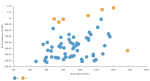# DCPY.ENVELOPE(contamination, columns)

Elliptic Envelope is a multivariate outlier detection technique, which strongly assumes Gaussian distribution of underlying data. This assumption is used to identify outlying samples using robust covariance estimation.

###### Parameters
• contamination – Approximate proportion of outliers in the dataset, which is used as a threshold for the decision function, float (0;1) (default 0.1).

• columns – Dataset columns or custom calculations.

Example: DCPY.ENVELOPE(0.1, sum([Gross Sales]), sum([No of customers])) used as a calculation for the Color field of the Scatterplot visualization.###### Input data
• Numeric variables are automatically scaled to zero mean and unit variance.

• Character variables are transformed to numeric values using one-hot encoding.

• Dates are treated as character variables, so they are also one-hot encoded.

• Size of input data is not limited, but many categories in character or date variables increase rapidly the dimensionality.

• Rows that contain missing values in any of their columns are dropped.

###### Result
• Column of values 1 corresponding to inlier, and -1 corresponding to outlier.
• Rows that were dropped from input data due to containing missing values have missing value instead of assigned inlier/outlier value.
###### Key usage points
• Data needs to be Gaussian distributed, otherwise it losses reliability.

• Works well when the dataset does not contain many variables.

###### Example

For the whole list of algorithms, see Data science built-in algorithms.